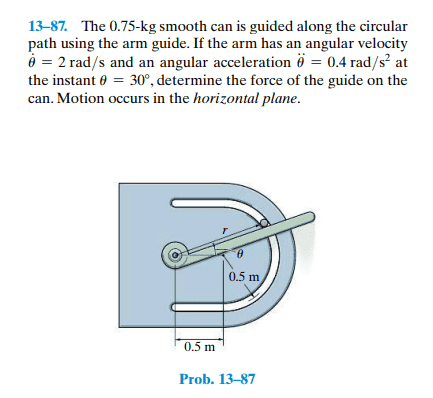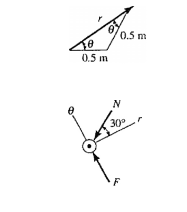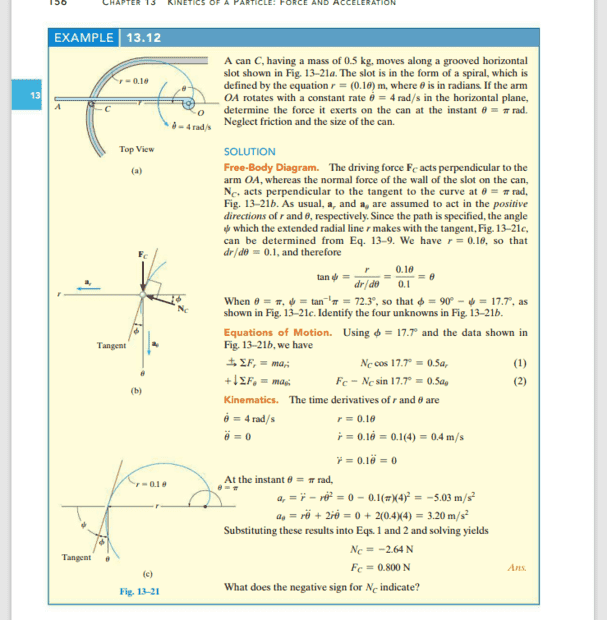# Angle between normal force and radial line for cylindrical coordinates

simphys
Homework Statement:
The 0.75-kg smooth can is guided along the circular
path using the arm guide. If the arm has an angular velocity ##\theta^. = 2rad/s## and an angular acceleartion of 0.4 rad/s^2 at the instant ##\theta = 30degrees##, determine the force of the guide on the can. Motion occurs in the horizontal plane.
Relevant Equations:
cylindrical stuffso I was wondering. there is this normal force on the can from the path. And there's this formula to find the angle between the radial line and the tangent or also between the normal force and either the radial or theta axis. the formula is ##\psi = r/dr/d\theta##. The thing is that here they have just used ##\theta = 30degrees## for some reason and I can't quite see why they use 30 degrees as being the angle between the axis and the normal force instead of the psi angle. Could someone clarify this please?

simphys
for more clarity:here, 30 degrees is used, but why is that?

Gold Member
for more clarity:
View attachment 319772
here, 30 degrees is used, but why is that?
because the net force you have labeled as ##N## (without friction) always acts toward the paths center.

•simphys
simphys
because the net force you have labeled as ##N## (without friction) always acts toward the paths center.
what do you mean? the path's center is not in the pin of the arm guide right? and the force F is the force exerted by the arm guide on the can/ball or whatever.

Gold Member
what do you mean? the path's center is not in the pin of the arm guide right? and the force F is the force exerted by the arm guide on the can/ball or whatever.
for the circular arc the normal force from the guide path is directed toward the center of the path ( ie the center of the circle - not the center of the pin for the arm ). It must be normal to the tangent at the point of interest.

•simphys
simphys
for the circular arc the normal force from the guide path is directed toward the center of the path ( ie the center of the circle - not the center of the pin for the arm ). It must be normal to the tangent at the point of interest.
yes yes of coursre that I know, and that is why I am asking why I can't use the formula to determine the angle between the radial line r shown and the normal force. Instead they use 30 degrees here, but how do you determine that?

Gold Member
yes yes of coursre that I know, and that is why I am asking why I can't use the formula to determine the angle between the radial line r shown and the normal force. Instead they use 30 degrees here, but how do you determine that?
I'm sorry I don't think I understand your question. They are telling you its 30 deg ( ## \theta = 30^{\circ} ## ) at the instant shown. Because there is no friction ( and its an isosceles triangle), that angle between ##r## and ##N## must be ##\theta##.

simphys
I'm sorry I don't think I understand your question. They are telling you its 30 deg ( ## \theta = 30^{\circ} ## ) at the instant shown. Because there is no friction ( and its an isosolies triangle), that angle between ##r## and ##N## must be ##\theta##.
well, so if you look at this example the angle psi, the formula I mentioned above is used for the normal force angle.Gold Member
well, so if you look at this example the angle psi, the formula I mentioned above is used for the normal force angle.
View attachment 319773

I believe you can use it?

•simphys
simphys
I believe you can use it?
actually you might be right.. I got something in the 40's, but I had the wrong equation for r=f(##\theta##) one sec let me try again.

simphys
actually you might be right.. I got something in the 40's, but I had the wrong equation for r=f(##\theta##) one sec let me try again.
nope... I am getting -40.89

simphys
Its says this is in the horizontal plane, so this is a top view (gravity is acting into the page)
oh, that is what they mean.., okay thanks a lot. I thought that it was downwards or something.

Gold Member
nope... I am getting -40.89

•simphys
simphys
##r = cos(\theta)## and for the given, r = 0.866 and for rdot --> ##rdot = sin(\theta) * \theta(dot)## = -1

Gold Member
##r = cos(\theta)## and for the given, r = 0.866 and for rdot --> ##rdot = sin(\theta) * \theta(dot)## = -1
$$\frac{dr}{d \theta} \neq \dot r$$

•simphys
simphys
$$\frac{dr}{d \theta} \neq \dot r$$

simphys
how come is it not?

simphys
oh dear......

Gold Member
how come is it not?
becuase ## \dot r = \frac{dr}{dt}##

simphys
I get - 60 degrees which indeed makes it 30 degrees now..... thanks a lot!! Need to watch out on those two mistakes I just made here.

•erobz